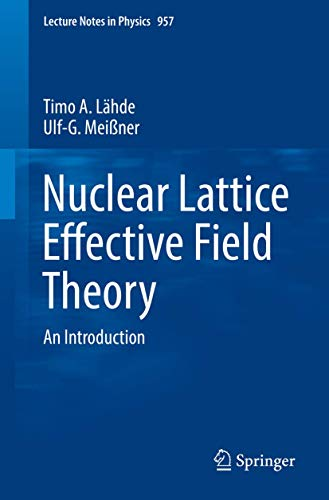• Free Shipping on all orders in Australia
• Over 7 million books in stock
• Proud to be B-Corp
• We aim to be carbon neutral by 2022
• Over 120,000 Trustpilot reviews
Item 1 of 0# Nuclear Lattice Effective Field Theory by Timo A. Lahde

Condition - New
\$109,09
Only 2 left

## Summary

This primer begins with a brief introduction to the main ideas underlying Effective Field Theory (EFT) and describes how nuclear forces are obtained from first principles by introducing a Euclidean space-time lattice for chiral EFT.

## Nuclear Lattice Effective Field Theory Summary

### Nuclear Lattice Effective Field Theory: An Introduction by Timo A. Lahde

This primer begins with a brief introduction to the main ideas underlying Effective Field Theory (EFT) and describes how nuclear forces are obtained from first principles by introducing a Euclidean space-time lattice for chiral EFT. It subsequently develops the related technical aspects by addressing the two-nucleon problem on the lattice and clarifying how it fixes the numerical values of the low-energy constants of chiral EFT. In turn, the spherical wall method is introduced and used to show how improved lattice actions render higher-order corrections perturbative. The book also presents Monte Carlo algorithms used in actual calculations.

In the last part of the book, the Euclidean time projection method is introduced and used to compute the ground-state properties of nuclei up to the mid-mass region. In this context, the construction of appropriate trial wave functions for the Euclidean time projection is discussed, as well as methods for determining the energies of the low-lying excitations and their spatial structure. In addition, the so-called adiabatic Hamiltonian, which allows nuclear reactions to be precisely calculated, is introduced using the example of alpha-alpha scattering. In closing, the book demonstrates how Nuclear Lattice EFT can be extended to studies of unphysical values of the fundamental parameters, using the triple-alpha process as a concrete example with implications for the anthropic view of the Universe.

Nuclear Lattice Effective Field Theory offers a concise, self-contained, and introductory text suitable for self-study use by graduate students and newcomers to the field of modern computational techniques for atomic nuclei and nuclear reactions.

## Nuclear Lattice Effective Field Theory Reviews

"Nuclear Lattice Effective Field Theory ... is a great practical advantage to the reader. ... Lahde and Meissner's helpful primer has the potential to stimulate increased efforts by serving newcomers as an essential guide to the field." (Ruprecht Machleidt, Physics Today, Vol. 72 (10), October, 2019)

Introduction to Effective Field Theory.- Nuclear Forces in Chiral EFT.- Lattice Formulations.- Lattice Chiral Effective Field Theory.- Two and Three Nucleons on the Lattice.- Lattice Monte Carlo.- LIght and Medium-Mass Nuclei on the Lattice.- Further Developments.- Notations and Conventions.- Basics of the Nucleon-Nucleon Interaction.- Study of Rotational Symmetry Breaking Effects in an A Cluster Model.- Monte Carlo Sampling.- Hybrid Monte Carlo Action and Force.- Monte Carlo Calculation of Observables.-

NLS9783030141875
9783030141875
303014187X
Nuclear Lattice Effective Field Theory: An Introduction by Timo A. Lahde
New
Paperback
Springer Nature Switzerland AG
2019-05-08
396
N/A
Book picture is for illustrative purposes only, actual binding, cover or edition may vary.
This is a new book - be the first to read this copy. With untouched pages and a perfect binding, your brand new copy is ready to be opened for the first time